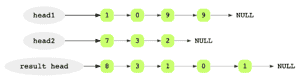# Add Two Integers

## Problem Statement

Given the head pointers of two linked lists where each linked list represents an integer number (each node is a digit), add them and return the resulting linked list. Here, the first node in a list represents the least significant digit.• Handle carry

## Try it yourself

LinkedListNode* add_integers(    LinkedListNode* integer1,     LinkedListNode* integer2) {  //TODO: Write - Your - Code  return integer1;}

## Solution

// assuming both integers are stored in a linked list// e.g. 415 is stored as 5->1->4// 32 is stored as 2->3LinkedListNode* add_integers(    LinkedListNode* integer1,     LinkedListNode* integer2) {  LinkedListNode* result = nullptr;  LinkedListNode* last = nullptr;  int carry = 0;    while (      integer1 != nullptr ||      integer2 != nullptr ||      carry > 0) {    int first =         (integer1 == nullptr ? 0 : integer1->data);    int second =         (integer2 == nullptr ? 0 : integer2->data);    int sum = first + second + carry;    LinkedListNode* pNew =           new LinkedListNode(sum % 10);        carry = sum / 10;    if (result == nullptr) {      result = pNew;    } else {      last->next = pNew;    }    last = pNew;        if (integer1 != nullptr) {      integer1 = integer1->next;    }        if (integer2 != nullptr) {      integer2 = integer2->next;    }  }    return result;}int main(int argc, char* argv[]) {	vector<int> v1 = {1, 2, 3}; // 321  vector<int> v2 = {1, 2}; // 21    LinkedListNode* first = LinkedList::create_linked_list(v1);  LinkedListNode* second = LinkedList::create_linked_list(v2);  // sum should be 321 + 21 = 342 => 2->4->3  LinkedListNode* result = add_integers(first, second);  vector<int> r = {2, 4, 3}; // 342  LinkedListNode* expected = LinkedList::create_linked_list(r);  assert(LinkedList::is_equal(result, expected));  cout << endl << "First:";  LinkedList::display(first);  cout << endl << "Second:";  LinkedList::display(second);  cout << endl << "Result:";  LinkedList::display(result);  result = add_integers(first, nullptr);  assert(LinkedList::is_equal(result, first));  result = add_integers(nullptr, second);  assert(LinkedList::is_equal(result, second));}

## Solution Explanation

### Runtime Complexity

Linear, O(n)

Runtime complexity is based on the length of the linked lists.

Linear, O(n)

### Solution Breakdown

For a better understanding of the problem, let’s take a look at an example. Suppose we want to add the integers 9901 and 237. The result of this addition would be 10138.

The integers are stored inverted in the linked lists to make the addition easier. Now, the most significant digit of the number is the last element of the linked list. For the addition, we’ll start from the heads of the two linked lists. At each iteration, we add the current digits of the two lists and insert a new node with the resulting digit at the tail of the result linked list. We’ll also need to maintain carry at each step. We’ll keep doing this for all digits in both the linked lists. If one of the linked lists ends sooner, we’ll continue with the other linked list. Once both of the linked lists are exhausted, and no carry is left to be added, the algorithm will terminate.

Learn in-demand tech skills in half the time